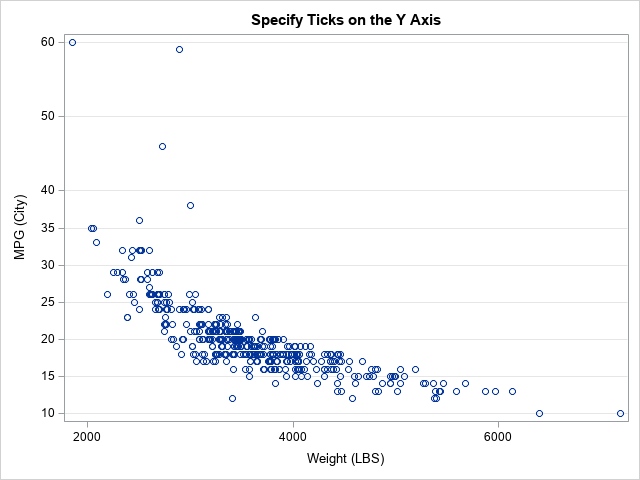On this blog, I write about a diverse set of topics that are relevant to statistical programming and data visualization. In a previous article, I presented some of the most popular blog posts from 2021. The most popular articles often deal with elementary or familiar topics that are useful to almost every data analyst. However, I also write articles that discuss more advanced topics. Did you make a New Year's resolution to learn something new this year? Here is your chance! The following articles deserve a second look. I have grouped them into four categories: SAS programming, statistics, optimization, and data simulation.

### SAS programming

For years, I've been writing articles about how to accomplish tasks in Base SAS. In addition, I now write about how to program basic tasks in SAS Viya.Use the DOLIST Syntax to Specify Tick Marks on a Graph

### Probability and statistics

Probability and statistics provide the theoretical basis for modern data analysis. You cannot understand data science, machine learning, or artificial intelligence without understanding the basic principles of statistics. Most readers are familiar with common probability distributions, Pearson correlation, and least-squares regression. The following articles discuss some of the lesser-known statistical cousins of these topics:

• The inverse gamma distribution: To use any probability distribution, you need to know four essential functions: the density, the cumulative probability, the quantiles, and how to generate random variates. You might be familiar with the gamma distribution, but what is the inverse gamma distribution and how do you define the four essential functions in SAS? Similarly, what is the generalized gamma distribution?
• The Hoeffding D statistic: The Hoeffding D statistic measures the association between two variables. How do you compute the Hoeffding D statistic in SAS, and how do you interpret the results?
• Weibull regression: In ordinary least squares regression, the response variable is assumed to be modeled by a set of explanatory variables and normally distributed errors. If the error terms are Weibull distributed, you can estimate parameters for the Weibull distribution as part of a regression analysis, but you need to transform the regression estimates to obtain the usual Weibull parameters.

### Optimization

Optimization is at the heart of many statistical techniques, such as maximum likelihood estimation. But sometimes you need to solve a "pure" optimization problem. SAS supports many methods for solving optimization problems:

### Multivariate simulation and bootstrapping

It is straightforward to simulate univariate data. It is harder to simulate multivariate data while preserving important relations between the variables, such as correlation. Similarly, it can be challenging to analyze the bootstrap distribution of a multivariate statistic, such as a correlation matrix: